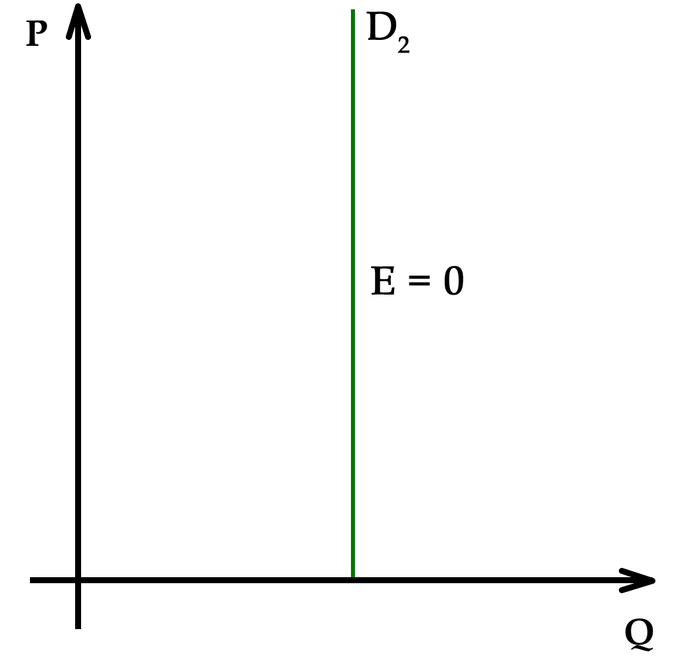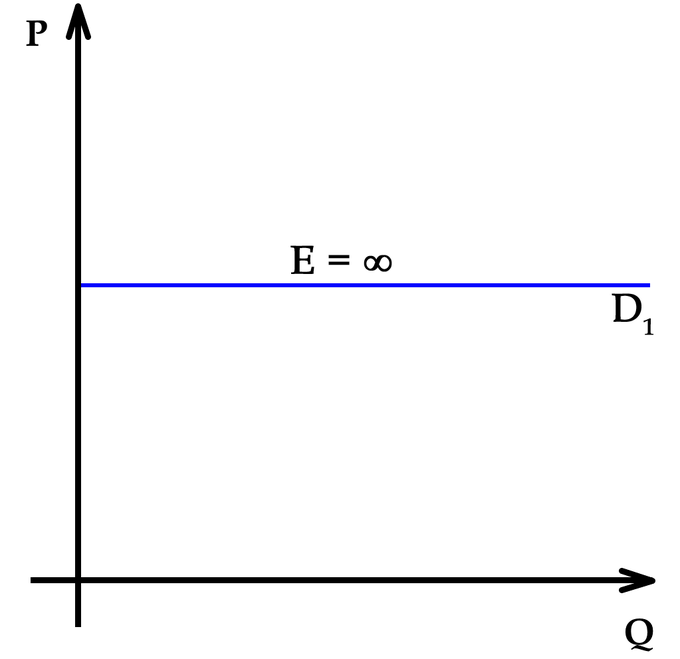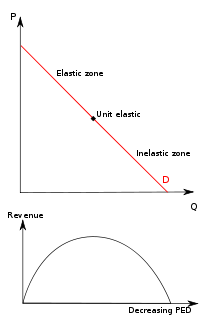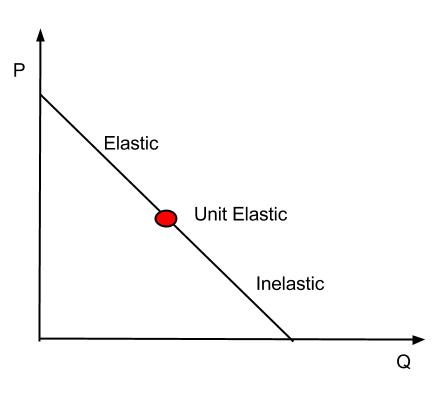## Defining Price Elasticity of Demand

The price elasticity of demand (PED) measures the change in demand for a good in response to a change in price.

### Learning Objectives

Define the price elasticity of demand.

### Key Takeaways

#### Key Points

• The PED is the percentage change in quantity demanded in response to a one percent change in price.
• The PED coefficient is usually negative, although economists often ignore the sign.
• Demand for a good is relatively inelastic if the PED coefficient is less than one (in absolute value).
• Demand for a good is relatively elastic if the PED coefficient is greater than one (in absolute value).
• Demand for a good is unit elastic when the PED coefficient is equal to one.

#### Key Terms

• elastic: Demand for a good is elastic when a change in price has a relatively large effect on the quantity of the good demanded.
• Unit Elastic: Demand for a good is unit elastic when the percentage change in quantity demanded is equal to the percentage change in price.
• inelastic: Demand for a good is inelastic when a change in price has a relatively small effect on the quantity of the good demanded.

The price elasticity of demand (PED) is a measure that captures the responsiveness of a good’s quantity demanded to a change in its price. More specifically, it is the percentage change in quantity demanded in response to a one percent change in price when all other determinants of demand are held constant.

The formula for the coefficient of PED is:

$PED\quad =\quad \frac { \%\quad change\quad in\quad quantity\quad demanded }{ \%\quad change\quad in\quad price\quad } \\ \\$

The law of demand states that there is an inverse relationship between price and demand for a good. As a result, the PED coefficient is almost always negative. However, economists tend to ignore the sign in everyday use. Only goods that do not conform to the law of demand, such as Veblen and Giffen goods, have a positive PED.

The numerical values for the PED coefficient could range from zero to infinity. In general, the demand for a good is said to be inelastic (or relatively inelastic) when the PED is less than one (in absolute value): that is, changes in price have a less than proportional effect on the quantity of the good demanded. The demand for a good is said to be elastic (or relatively elastic) when its PED is greater than one. In this case, changes in price have a more than proportional effect on the quantity of a good demanded.

A PED coefficient equal to one indicates demand that is unit elastic; any change in price leads to an exactly proportional change in demand (i.e. a 1% reduction in demand would lead to a 1% reduction in price).

A PED coefficient equal to zero indicates perfectly inelastic demand. This means that demand for a good does not change in response to price.Perfectly Inelastic Demand: When demand is perfectly inelastic, quantity demanded for a good does not change in response to a change in price.

Finally, demand is said to be perfectly elastic when the PED coefficient is equal to infinity. When demand is perfectly elastic, buyers will only buy at one price and no other.Perfectly Elastic Demand: When the demand for a good is perfectly elastic, any increase in the price will cause the demand to drop to zero.

## Measuring the Price Elasticity of Demand

The price elasticity of demand (PED) is calculated by dividing the percentage change in quantity demanded by the percentage change in price.

### Learning Objectives

Calculate the own-price elasticity of demand

### Key Takeaways

#### Key Points

• PED captures the change in quantity demanded in response to a change in the good’s own price (as opposed to the price of some other good).
• The formula for price elasticity yields a value that is negative, pure, and ranges from zero to negative infinity.
• The result provided by the formula will be accurate only if the changes in price and quantity demanded are small.

#### Key Terms

• Own-price elasticity of demand: Responsiveness of quantity demanded to a change in the good’s own price
• Cross-price elasticity of demand: Measures the responsiveness of the demand for a good to a change in the price of another good.

The price elasticity of demand (PED) captures how price-sensitive consumers are for a given product or service by measuring the responsiveness of quantity demanded to changes in the good’s own price. This is in contrast to measuring the responsiveness of the good’s demand to a change in price for some other good (a complement or substitute), which is called the cross-price elasticity of demand. The own-price elasticity of demand is often simply called the price elasticity.

The following formula is used to calculate the own-price elasticity of demand:

$Elasticity\quad =\quad \frac { \%\quad Change\quad in\quad Quantity\quad Demanded\quad }{ \%\quad Change\quad in\quad Price }$

The formula above usually yields a negative value because of the inverse relationship between price and quantity demanded. However, economists often disregard the negative sign and report the elasticity as an absolute value. For example, if the price of a good increases by 5 percent and the quantity demanded decreases by 5 percent, then the elasticity at the initial price and quantity is -5%/5% = -1. This number is likely to be reported simply as 1.Sale: There is an inverse relationship between price and quantity demanded, so the elasticity coefficient is almost always negative.

There are a few other important points to note about the coefficient value provided by this formula. First, the elasticity coefficient is a pure number, meaning that it does not have units of measurement associated with it. Second, the coefficient value can range from zero to negative infinity. Finally, the result provided by the formula will be accurate only when the changes in price and quantity are small. The result will be less accurate when the changes are large.

Since PED is based off of percent changes, the starting nominal quantity and price matter. At low prices and high quantities, the PED is therefore more inelastic. For example, a drop in the price of $1 from a starting price of$100 is a 1% drop, but if the starting price is \$10, it is a 10% drop. Similarly, at high prices and low quantities, PED is more elastic.Price Elasticity of Demand and Revenue: PED is based off of percent changes, so the starting nominal values of price and quantity are significant.

## Interpretations of Price Elasticity of Demand

The price elasticity of demand (PED) explains how much changes in price affect changes in quantity demanded.

### Learning Objectives

Describe the relationship between price elasticity and the shape of the demand curve.

### Key Takeaways

#### Key Points

• Elastic PED can be interpreted as consumers being very sensitive to changes in price.
• Inelastic PED can be interpreted as consumes being insensitive to changes in price.
• Firms use PED to figure out how to change their prices in order to increase revenue.
• PED varies along a straight demand curve.

#### Key Terms

• Price elasticity of demand: The percent change in quantity demanded due to a 1% change in price.

The price elasticity of demand (PED) is a measure of the responsiveness of the quantity demanded of a good to a change in its price. It can be calculated from the following formula:

$\frac{\%Change \; in \; Quantity \; Demanded}{\%Change \; in \; Price}$

When PED is greater than one, demand is elastic. This can be interpreted as consumers being very sensitive to changes in price: a 1% increase in price will lead to a drop in quantity demanded of more than 1%.

When PED is less than one, demand is inelastic. This can be interpreted as consumers being insensitive to changes in price: a 1% increase in price will lead to a drop in quantity demanded of less than 1%.

The effect of price changes on total revenue PED may be important for businesses attempting to distinguish how to maximize revenue For example, if a business finds out its PED is very inelastic, it may want to raise its prices because it knows that it can sell its products for a higher price without losing many sales. Conversely, if a business finds that its PED is very elastic, it may wish to lower its prices. This would allow the business to dramatically increase the number of units sold without losing much revenue per unit.

There are two notable cases of PED. The first is when demand is perfectly elastic. Perfectly elastic demand is represented graphically as a horizontal line. In this case, any increase in price will lead to zero units demanded.Perfectly Elastic Demand: Perfectly elastic demand is represented graphically by a horizontal line. In this case the PED value is the same at every point of the demand curve.

The second is perfectly inelastic demand. Perfectly inelastic demand is graphed as a vertical line and indicates a price elasticity of zero at every point of the curve. This means that the same quantity will be demanded regardless of the price.Perfectly Inelastic Demand: Perfectly inelastic demand is graphed as a vertical line. The PED value is the same at every point of the demand curve.

Since PED is measured based on percent changes in price, the nominal price and quantity mean that demand curves have different elasticities at different points along the curve. Elasticity along a straight line demand curve varies from zero at the quantity axis to infinity at the price axis. Below the midpoint of a straight line demand curve, elasticity is less than one and the firm wants to raise price to increase total revenue. Above the midpoint, elasticity is greater than one and the firm wants to lower price to increase total revenue. At the midpoint, E1, elasticity is equal to one, or unit elastic.Elasticity and the Demand Curve: The price elasticity of demand for a good has different values at different points on the demand curve.

## Determinants of Price Elasticity of Demand

A good’s price elasticity of demand is largely determined by the availability of substitute goods.

### Learning Objectives

Explain how a good’s price elasticity of demand may be different in the short term than in the long term

### Key Takeaways

#### Key Points

• A good with more close substitutes will likely have a higher elasticity.
• The higher the percentage of a consumer’s income used to pay for the product, the higher the elasticity tends to be.
• For non-durable goods, the longer a price change holds, the higher the elasticity is likely to be.
• The more necessary a good is, the lower the price elasticity of demand.

#### Key Terms

• Substitute Good: A good that fulfills a consumer need in a way that is similar to another good.

The price elasticity of demand (PED) is a measure of how much the quantity demanded changes with a change in price. The PED for a given good is determined by one or a combination of the following factors:

• Availability of substitute goods: The more possible substitutes there are for a given good or service, the greater the elasticity. When several close substitutes are available, consumers can easily switch from one good to another even if there is only a small change in price. Conversely, if no substitutes are available, demand for a good is more likely to be inelastic.
• Proportion of the purchaser’s budget consumed by the item: Products that consume a large portion of the purchaser’s budget tend to have greater elasticity. The relative high cost of such goods will cause consumers to pay attention to the purchase and seek substitutes. In contrast, demand will tend to be inelastic when a good represents only a negligible portion of the budget.
• Degree of necessity: The greater the necessity for a good, the lower the elasticity. Consumers will attempt to buy necessary products (e.g. critical medications like insulin) regardless of the price. Luxury products, on the other hand, tend to have greater elasticity. However, some goods that initially have a low degree of necessity are habit-forming and can become “necessities” to consumers (e.g. coffee or cigarettes).
• Duration of price change: For non-durable goods, elasticity tends to be greater over the long-run than the short-run. In the short-term it may be difficult for consumers to find substitutes in response to a price change, but, over a longer time period, consumers can adjust their behavior. For example, if there is a sudden increase in gasoline prices, consumers may continue to fuel their cars with gas in the short-run, but may lower their demand for gas by switching to public transportation, carpooling, or buying more fuel-efficient vehicles over a longer period of time. However, this tendency does not hold for consumer durables. The demand for durables (cars, for example) tends to be less elastic, as it becomes necessary for consumers to replace them with time.
• Breadth of definition of a good: The broader the definition of a good, the lower the elasticity. For example, potato chips have a relatively high elasticity of demand because many substitutes are available. Food in general would have an extremely low PED because no substitutes exist.
• Brand loyalty: An attachment to a certain brand (either out of tradition or because of proprietary barriers) can override sensitivity to price changes, resulting in more inelastic demand.